# Physics 12th Electric Charge and Electric Field (12th and IIT,AIEEE Notes)

Physics 12th Electric Charge and Electric Field (12th and IIT,AIEEE Notes)

### 1. Electric Charge

• Electric charge is a fundamental property like mass, length etc associated with elementary particles for example electron, proton and many more.
• Electric charge is the property responsible for electric forces which acts between nucleus and electron to bind the atom together.
• Charges are of two kinds
(i) negative charge
(ii) positive charge
• Electrons are negatively charged particles and protons, of which nucleus is made of, are positively charged particles. Actually nucleus is made of protons and neutrons but neutrons are uncharged particles.
• electric force between two electrons is same as electric force between two protons kept at same distance apart i. e., both set repel each other but electric force between an electron and proton placed at same distance apart is not repulsive but attractive in nature.

Conclusion
(a) Like charges repel each other(b) Unlike charges attract each other• Assignment of negative charge on electron and positive charge on proton is purely conventional , it does not mean that charge on electron is less than that on proton.
• Importance of electric forces is that it encompasses almost each and every field associated with our life; being it matter made up of atoms or molecules in which electric charges are exactly balanced or adhesive forces of glue associated with surface tension, all are electric in nature.

Unit

• Charge on a system can be measured by comparing it with the charge on a standard body.
• SI unit of charge is Coulomb written as C.
• 1 Coulomb is the charge flowing through the wire in 1 second if the electric current in it is 1A.
• Charge on electron is -1.602 × 10 -19 C and charge on proton is positive of this value.

### 2. Basic properties of electric charge

• Charges adds up like real numbers i. e., they are Scalars more clearly if any system has n number of charges q1, q2, q3, qn then total charge of the system is
q = q1 + q2 + q3 + ................ qn
• Proper sign have to be used while adding the charges for example if
q1 = +1C
q2 = -2C
q3 = +4C
then total charge of the system is
q = q1 + q2 + q3
q = (+1) + (-2) + (+4) C
q = (+3) C
(ii) Charge is conserved

• Charge of an isolated system is conserved.
• Chage can not be created or destroyed but charged particles can be created or destroyed.

(iii) Quantization of charge

• All free charges are integral multiples of a unit of charge e, where e = -1.602 × 10 -19 C i. e., charge on an electron or proton.
• Thus charge q on a body is always denoted by
q = ne
where n = any integer positive or negative
• 3. Frictional Electricity

• If we pass a comb through hairs, comb becomes electrically charged and can attract small pieces of paper.
• Many such solid materials are known which on rubbing attract light objects like light feather, bits of papers, straw etc.
• Explaination of appearance of electric charge on rubbing is simple.
• Material bodies consists of large number of electrons and protons in equal number and hence is in neutral in their normal state. But when the body is rubbed for example when a glass rod is rubbed with silk cloth, electrons are transferred from glass rod to silk cloth. The glass rod becomes positively charged and the silk cloth becomes negatively charged as it recieves extra electrons from the glass rod.
• In this case rod after rubbing, comb after passing through dry hairs becomes electrified and these are the example of frictional electricity.

4. Coulumb's law

• Coulomb's law is the law of forces between electric charges.
Statement
" It states that two stationary point charges q1 and q2 repel or attract each other with a force F which is directly proportional to the product of charges and inversly proportional to the square of distance between them."

This dependence can be expressed by writing
 F∝ q1q2 r2
(1)
• These forces are attractive for unlike charges and repulsive for like charges .
• We now try to express Coulomb's law in vector form for more clearity of magnitude and direction of forces.
• Consider two point charges q1 and q2 at points with position vector r1 and r2 with respect to the originvector r21r2 - r1 is the difference between r2 and r1 and the distance of separation r is the magnitude of vector r21.
pointwise it can be written as
r1 = position vector of charge q1 with respect to origin
r2 = position vector of charge q2 with respect to origin
r21 = vector from 1 to 2 (r2 - r1)
r12 = -r21 = vector from 2 to 1 (r1 r2)
= r12 = r21 = distance between 1 and 2.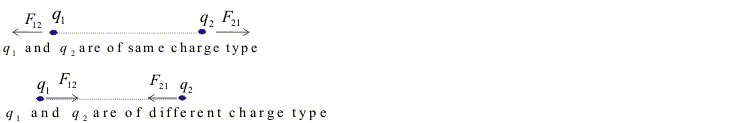Coulomb's law can then be expressed as
F21 = force on q2 due to q1
 F21= kq1q2r21 r3
(2a)
and, F12 = force on q1 due to q2
 F12= -F21 kq1q2r12 r3
(2b)

Special Case
• for simplicity we can choose q
1
being placed at origin

r1
= 0

and if we write
r2
=
r
the position vector of q
2
then

F21
= force on q
2
due to q
1
 F21= kq1q2r r3
(3a)

F12
= force on q
1
due to q
2
 F12= - kq1q2r r3
(3b)

unit vector r
ˆ21
and r
ˆ

12
can be defined as

rˆ21
=
r21
/r directed from q
1
to q
2
rˆ12
=
r12
/r directed from q
2
to q
1
(4)

= -
r21
/r

force can now be written in terms of unit vector given as follows

 F21= kq1q2rˆ21 r2
(5a)
 F12= kq1q2rˆ12 r2
(5b)

from this we can immidiately find factors giving magnitude and the directions

• in equation (2) we find a positive constant K and experimentally found value of k is
K = 8.98755 × 10 9 Nm2/C2
K ≅ 9 × 10 9 Nm2/C2
sometimes K is written as 1/4π ε0 where ε0 is the permittivity of the vaccum whose value is
K = 1/4πε0
0 = 9 × 10 -12 C2/Nm2)
• 5. Principle Of Superposition

• Coulomb's law gives the electric force acting between two electric charges.
• Principle of superposition gives the method to find force on a charge when system consists of large number of charges.
• According to this principle when a number of charges are interacting the total force on a given charge is vector sum of forces exerted on it by all other charges.
• This principle makes use of the fact that the forces with which two charges attract or repel one another are not affected by the presence of other charges.
• If a system of charges has n number of charges say q1, q2, ...................., qn, then total force on charge q1 according to principle of superposition is
F = F12 + F13 + .................................. F1n
Where F12 is force on q1 due to q2 and F13 is force on q1 due to q3 and so on.• F12F13, .................. F1n can be calculated from Coulomb's law i. e.
 F12= kq1q2rˆ12 4πε0(r12)2
to,
 F1n= kq1q2rˆ1n 4πε0(r1n)2
• The total force F1 on the charge q1 due to all other charges is the vector sum of the forces F12F13, ................................. F1n.
F1 = F12 + F13 + ..................................• The vector sum is obtained by parellogram law of addition of vector.
• Similarly force on any other charge due to remaining charges say on q2, q3 etc. can be found by adopting this method.

6. Electric Field

• Electrical interaction between charged particles can be reformulated using the concept of electric field.
• To understand the concept consider the mutual repulsion of two positive charged bodies as shown in fig (a)• Now if remove the body B and label its position as point P as shown in fig (b), the charged body A is said to produce an electric field at that point (and at all other points in its vicinity)
• When a body B is placed at point P and experiences force F, we explain it by a point of view that force is exerted on B by the field not by body A itself.
• The body A sets up an electric field and the force on body B is exerted by the field due to A.
• An electric field is said to exists at a point if a force of electric origin is exerted on a stationary charged (test charge) placed at that point.
• If F is the force acting on test charge q placed at a point in an electric field then electric field at that point is
E = F/q
or F = qE
• Electric field is a vector quantity and since F = qE the direction of E is the direction of F.
• Unit of electric field is (N.C-1)
Q. Find the dimensions of electric field
Ans. [MLT-3A-1]

7. Calculation of Electric Field
• In previous section we studied a method of measuring electric field in which we place a small test charge at the point, measure a force on it and take the ratio of force to the test charge.
• Electric field at any point can be calculated using Coulomb's law if both magnitude and positions of all charges contributing to the field are known.
• To find the magnitude of electric field at a point P, at a distance r from the point charge q, we imagine a test charge q'to be placed at P. Now we find force on charge q' due to q through Coulomb's law.
 F= qq' 4πε0r2
electric field at P is   E= q 4πε0r2
The direction of the field is away from the charge q if it is positive• Electric field for either a positive or negative charge in terms of unit vector r directed along line from charge q to point P is   E= qrˆ 4πε0r2
r = distance from charge q to point P.
• When q is negative , direction of E is towards q, opposite to r.

Electric Field Due To Multiple Charges
• Consider the number of point charges q1, q2,........... which are at distance r1P, r2P,................... from point P as shown in fig• The resultant electric field is the vector sum of individual electric fields as
E = E1P + E2P + .....................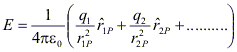This is also a direct result of principle of superposition discussed earlier in case of electric force on a single charge due to system of multiple charges.
• E is a vector quantity that varies from one point in space to another point and is determined from the position of square charges.
8. Electric Field Lines
• For a single positive point charge q, electric field is
 E= qrˆ 4πε0r2

now to get feel of this field one can sketch a few representative vectors as shown in fig below• Since electric field varies as inverse of square of the distance that points from the charge the vector gets shorter as you go away from the origin and they always points radially outwards.
• Connecting up these vectors to form a line is a nice way to represent this field .
• The magnitude of the field is indicated by the density of the field lines.
• Magnitude is strong near the center where the field lines are close togather, and weak farther out, where they are relatively apart.
• So, electric field line is an imaginary line drawn in such a way that it's direction at any point is same as the direction of field at that point.
• An electric field line is, in general a curve drawn in such a way that the tangent to it ateach point is the direction of net field at that point.
• Field lines of a single position charge points radially outwards while that of a negative charge are radially inwards as shown below in the figure.• Field lines around the system of two positive charges gives a different picture and describe the mutual repulsion between them.• Field lines around a system of a positive and negative charge clearly shows the mutual attraction between them as shown below in the figure.• Some important general properties of field lines are
1.Field lines start from positive charge and end on a negative charge.
2.Field lines never cross each other if they do so then at the point of intersection there will be two direction of electric field.
3.Electric field lines do not pass through a conductor , this shows that electric field inside a conductor is always zero.
4.Electric field lines are continuous curves in a charge free region.

9. Electric Flux

• Consider a plane surface of area ΔS in a uniform electric field E in the space.
• Draw a positive normal to the surface and θ be the angle between electric field E and the normal to the plane.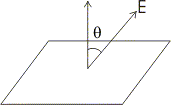• Electric flux of the electric field through the choosen surface is then
Δφ = E ΔS cosθ
• Corresponding to area ΔS we can define an area vector ΔS of magnitude ΔS along the positive normal. With this definition one can write electric flux as
Δφ = E . ΔS
• direction of area vector is always along normal to the surface being choosen.
• Thus electric flux is a measure of lines of forces passing through the surface held in the electric field.

Special Cases
• If E is perpendicular to the surface i. e., parallel to the area vector then θ = 0 and
Δφ = E ΔS cos0
• If θ = π i. e., electric field vector is in the direction opposite to area vector then
Δφ = - E ΔS
• If electric field and area vector are perpendicular to each other then θ = π/2 and Δφ = 0
• Flux is an scaler quantity and it can be added using rules of scaler addition.
• For calculating total flux through any given surface , divide the surface into small area elements. Calculate the flux at each area element and add them up.
• Thus total flux φ through a surface S is
φ ≅ ΣE.ΔS
• This quantity is mathematically exact only when you take the limit ΔS→0 and the sum in equation 3 is written as integral
φ = ∫ΣE.dS
9. Electric Dipole

• Electric dipole is a pair of equal and opposite charges, +q and -q, separated by some distance 2a.
• Total charge of the dipole is zero but electric field of the dipole is not zero as charges q and -q are separated by some distance and electric field due to them when added is not zero.

(A)Field of an electric dipole at points in equitorial plane
• We now find the magnitude and direction of electric field due to dipole.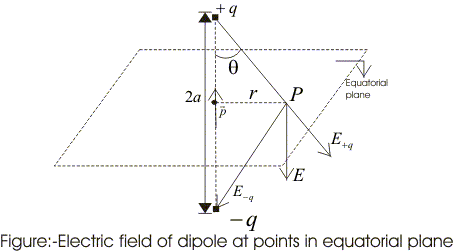• P point in the equitorial plane of the dipole at a distance r from the centre of the dipole. Then electric field due to +q and -q are   E-q= -qPˆ 4πε0(r2 + a2)
(1a)   E+q= qPˆ 4πε0(r2 + a2)
(1b)
and they are equal
= unit vector along the dipole axis (from -q to +q)
• From fig we can see the direction of E+q and E-q. Their components normal to dipole cancel away and components along the dipole add up.
• Dipole moment vector points from negative charge to positive charge so in vector form.
E = -(E+q + E-q) cos θ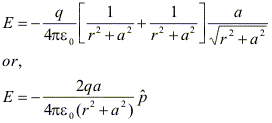(2)
At large distances (r>>a), above equation becomes
 E= -2qaPˆ 4πε0(r3)
(3)
and they are equal

(B) Field of an electric dipole for points on the axis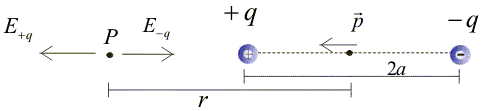• Let P be the point at a distance r from the centre of the dipole on side of charge q. as shown in the fig.
 E-q= -qPˆ 4πε0(r+a)2
(4a)
= unit vector along the dipole axis (from -q to +q)
also
 E+q= qPˆ 4πε0(r - a) 2

(4b)
• Total field at P is
E = E+q + E-q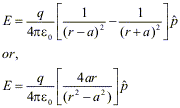(5)
for r>>a
 E= 4qaPˆ 4πε0r3

(6)
• For equation (3) and (6) charge q and dipole separation 2a appear in combination qa. This leads us to define dipole moment vector P of electric dipole. Thus, electric dipole moment P = q × 2a                                          (7)
• Unit of dipole moment is Coulomb's meter (Cm).
• In terms of electric dipole moment, field of a dipole at large distances becomes

(i) At point on equitorial plane (r>>a)
E = -P/4πε0r3
 E= -P 4πε0r3

(ii) At point on dipole axis (r>>a)
 E= 2P 4πε0r3
Note:-
(i) Dipole field at large distances falls off as 1/r3
(ii) Both the direction and magnitude of dipole an angle between dipole moment vector P and position vector r

(C) Dipole in a uniform external field
• Consider a dipole in a uniform electric field E whose direction makes an angle θ with dipole axis (line joining two charges)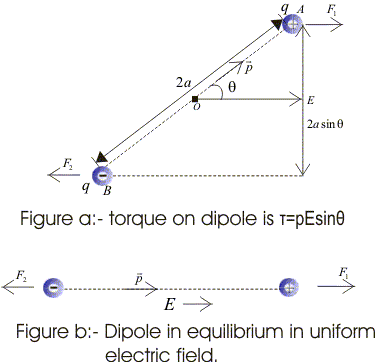• Force F1 of magnitude qE, acts on positive charge in direction of electric field and a force F2 of same magnitude acts on negative charge but it acts in direction opposite to F1.
• Resultant force on dipole is zero, but since two forces do not have same line of action they constitute a couple.
• We now calculate torque (r × F) of these forces about zero.

Torque of F1 about O is
τ1OB × F1
= q (OB × E)

Torque of F2 about O is
τ2 = OA × F2
= -q(OA × E)
= q(AO × E)

net torque acting on dipole is
τ τ1 + τ2
= q(OB+ AO) ×
= q (AB × E)

AB = 2a and p = 2qa (dipole moment)
τ =p × E
• Direction of torque is perpendicular to the plane containing dipole axis and electric field.
• Effect of torque is to rotate the dipole to a position in which dipole moment p is parallel to E the electric field vector is shown above in figure b and for uniform electric field dipole is in equilibrium in this position.
• magnitude of this torque is
τ = |τ| = pE sinθ
Examples

Question 1
Two point charges q1 and q2are located with points having position vectors r1 and r2
(1) Find the position vector r3 where the third charge q3 should be placed so that force acting on each of the three charges would be equal to zero.
(2) Find the amount of charge q3

Question 2
Consider a thin wire ring of radius R and carrying uniform charge density λ per unit length.
(1) Find the magnitude of electric field strength on the axis of the ring as a function of distance x from its centre.
(2) What would be the form of electric field function for x>>R.
(3) Find the magnitude of maximum strength of electric field.

Question 3
Two equally charged metal balls each of mass m Kg are suspended from the same point by two insulated threads of length l m long. At equilibrium, as a result of mutual separation between balls, balls are separated by x m. Determine the charge on each ball.

51 votes. Average: 3.33 / 5.